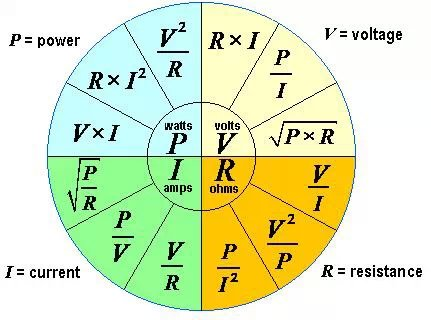##用户名 Email 自动登录 找回密码 密码 立即注册

# 功率、电压、电流、电阻换算表 换算公式daxiao 发表于 2015-3-21 23:48 | 显示全部楼层 |阅读模式
 换算公式 P（功率）=U（电压） × I（电流） 细节如下： ⑴串联电路 P（电功率）U（电压）I（电流）W（电功）R（电阻）T（时间） 电流处处相等 I1=I2=I 总电压等于各用电器两端电压之和 U=U1+U2 总电阻等于各电阻之和 R=R1+R2 U1：U2=R1：R2 总电功等于各电功之和 W=W1+W2 W1：W2=R1：R2=U1：U2 P1：P2=R1：R2=U1：U2 总功率等于各功率之和 P=P1+P2 ⑵并联电路 总电流等于各处电流之和 I=I1+I2 各处电压相等 U1=U1=U 总电阻等于各电阻之积除以各电阻之和 R=R1R2÷（R1+R2） 总电功等于各电功之和 W=W1+W2 I1：I2=R2：R1 W1：W2=I1：I2=R2：R1 P1：P2=R2：R1=I1：I2 总功率等于各功率之和 P=P1+P2 ⑶同一用电器的电功率 ①额定功率比实际功率等于额定电压比实际电压的平方 Pe/Ps=(Ue/Us)的平方 2．有关电路的公式 ⑴电阻 R ①电阻等于材料密度乘以（长度除以横截面积） R=密度×（L÷S） ②电阻等于电压除以电流 R=U÷I ③电阻等于电压平方除以电功率 R=UU÷P ⑵电功 W 电功等于电流乘电压乘时间 W=UIT（普式公式） 电功等于电功率乘以时间 W=PT 电功等于电荷乘电压 W=QT 电功等于电流平方乘电阻乘时间 W=I×IRT（纯电阻电路） 电功等于电压平方除以电阻再乘以时间 W=U?U÷R×T（同上） ⑶电功率 P ①电功率等于电压乘以电流 P=UI ②电功率等于电流平方乘以电阻 P=IIR（纯电阻电路） ③电功率等于电压平方除以电阻 P=UU÷R(同上) ④电功率等于电功除以时间 P＝W：T ⑷电热 Q 电热等于电流平方成电阻乘时间 Q=IIRt（普式公式） 电热等于电流乘以电压乘时间 Q=UIT=W（纯电阻电路 在同一电路中，导体中的电流跟导体两端的电压成正比，跟导体的电阻成反比,这就是欧姆定律 I=U/R 其中：I、U、R——三个量是属于同一部分电路中同一时刻的电流强度、电压和电阻。 由欧姆定律所推公式: 并联电路： 串联电路 I总=I1+I2 I总=I1=I2 U总=U1=U2 U总=U1+U2 1：R总=1：R1+1：R2 R总=R1+R2R I1：I2=R2：R1 U1：U2=R1：R2 R总=R1+R2：R1R2 R总=R1R2R3：R1R2+R2R3+R1R3 也就是说：电流＝电压÷电阻 或者 电压＝电阻×电流 流过电路里电阻的电流，与加在电阻两端的电压成正比，与电阻的阻值成反比。 功率、电压、电流、电阻换算表本版积分规则 回帖并转播 回帖后跳转到最后一页

Powered by 单片机教程网